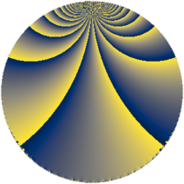# Properties

 Label 1344.4.uLevel $1344$ Weight $4$ Character orbit 1344.u Rep. character $\chi_{1344}(559,\cdot)$ Character field $\Q(\zeta_{4})$ Dimension $192$ Sturm bound $1024$

# Related objects

## Defining parameters

 Level: $$N$$ $$=$$ $$1344 = 2^{6} \cdot 3 \cdot 7$$ Weight: $$k$$ $$=$$ $$4$$ Character orbit: $$[\chi]$$ $$=$$ 1344.u (of order $$4$$ and degree $$2$$) Character conductor: $$\operatorname{cond}(\chi)$$ $$=$$ $$112$$ Character field: $$\Q(i)$$ Sturm bound: $$1024$$

## Dimensions

The following table gives the dimensions of various subspaces of $$M_{4}(1344, [\chi])$$.

Total New Old
Modular forms 1568 192 1376
Cusp forms 1504 192 1312
Eisenstein series 64 0 64

## Trace form

 $$192q + O(q^{10})$$ $$192q - 40q^{11} + 656q^{23} - 400q^{29} + 456q^{35} - 16q^{37} + 808q^{43} + 752q^{53} - 3288q^{67} - 896q^{71} - 15552q^{81} - 3496q^{91} + 360q^{99} + O(q^{100})$$

## Decomposition of $$S_{4}^{\mathrm{new}}(1344, [\chi])$$ into newform subspaces

The newforms in this space have not yet been added to the LMFDB.

## Decomposition of $$S_{4}^{\mathrm{old}}(1344, [\chi])$$ into lower level spaces

$$S_{4}^{\mathrm{old}}(1344, [\chi]) \cong$$ $$S_{4}^{\mathrm{new}}(112, [\chi])$$$$^{\oplus 6}$$$$\oplus$$$$S_{4}^{\mathrm{new}}(336, [\chi])$$$$^{\oplus 3}$$$$\oplus$$$$S_{4}^{\mathrm{new}}(448, [\chi])$$$$^{\oplus 2}$$